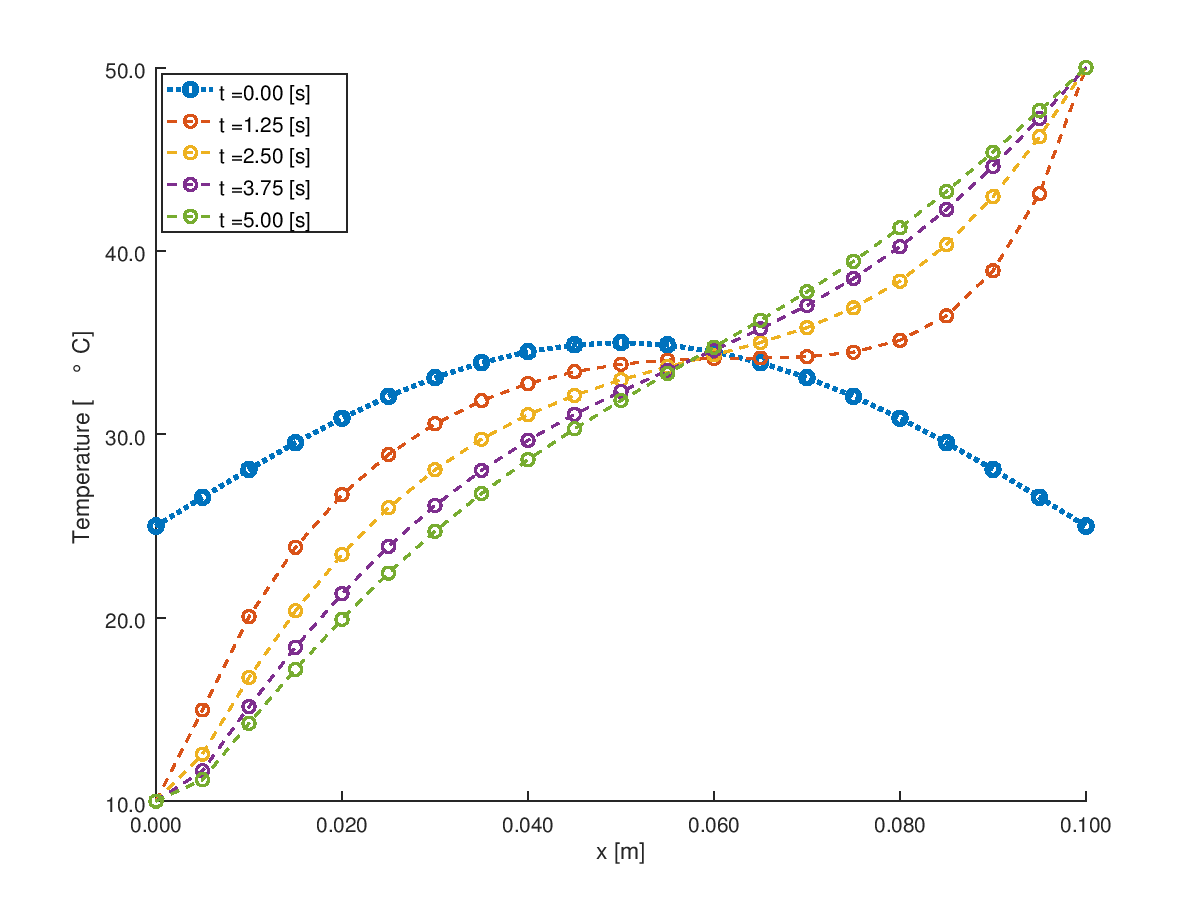• CFD, Fluid Flow, FEA, Heat/Mass Transfer

Octave Script

### Transient Conduction

OCTAVE is a free program highly compatible to MATLAB. It is a handy tool to solve differential equations numerically with built-in feature or use numerical methods dealing with inversion of matrices. A graphical plot of the results can be generated with ease.

The purpose of this calculation approach is to demonstrate the numerical technique and TDMA (Tri-Diagomal Matrix Algorithm) method to solve transient conduction. Various improvisation is possible in this script such as implementation of Heat Flux and Convective boundaries at the tip, incorporating variable cross-section area such as tapered rod and so on.

#### Temperature Time History Plots```% Solve 1D heat equation with Backward Time Centre Space scheme
%   d^2T     1      dT
%   ---- = ----- * ----
%   dx^2   alpha    dt
%
clear; clc;

% L        = length of the domain [m]
L        = 0.1;
% NN       = number of mesh nodes [number]
NN       = 21;

% nTime    = number of steps  [number], delta_t = tMax / nTime
nTime    = 4;
% tMax     = maximum time for the simulation [s]
tMax     = 5.0;

% alpha    = diffusion coefficient [m^2/s]
% Thermal conductivity [W/m.K]
k        = 150;
% Density [kg/m^3]
rho      = 2700;
% Specific heat capacity [J/kg.K]
Cp       = 450;
alpha    = k/rho/Cp;

% Specify initial conditions
dx  = L / (NN - 1);
x   = [0 : dx: L];
phi = 25 + 10 * sin(pi .* x / L);
% Specify boundary conditions
phi0     = 10;
phiL     = 50;

dt = tMax / nTime;

% Check for Courant number for numerical stability and bounded solution
CoNum = alpha * dt / dx^2;
%If (CoNum >= 2.0)
%  N = L / ((alpha * dt / 2.0)^0.5);
%	 NN = round(N);
%  dx = L / (NN - 1);
%endif

% --- Coefficients of the Tri-Diagonal System

% T^n(i-1) - 2T^n(i) + T^n(i+1)     1      T^n - T^[n-1](i)
% ----------------------------- = ----- *  --------------
%             dx^2                alpha         dt
%                     .--              --.
%   alpha             |  1     2*alpha   |          alpha            T^[n-1](i)
% - ----- T^n(i-1) +  | --- -  -------   |T^n(i) -  -----T^n(i+1) =  ----------
%    dx^2             |  dt     dx^2     |           dx^2                dt
%                     |__              __|
%
% -  C * T^n(i-1) +         A *          T^n(i) - B *   T^n(i+1) = d(i),  i = 1 ... NN
%
%  A* T^n(i) = B(i) * T(i+1) + C(i) * T(i-1) + d(i), i = 1 ... NN

% subdiagonal C: coefficients of phi(i-1)
B = (alpha/dx^2) * ones(NN,1);

% superdiagonal B: coefficients of phi(i+1)
C = B;

% diagonal A: coefficients of phi(i)
A = (1/dt) * ones(NN, 1) + 2 * B;

% constant term d
d = zeros(NN, 1);

% Plot temperature profile at t = 0 [s]
hold on;
plot(x, phi, "linestyle", ":", "linewidth", 2, "marker", "o");

for m = 1:nTime
% Tridiagonal Matrix Algorithm [Ref: S. V. Patankar]
% If T(1) is specified
A(1) = 1;     B(1) = 0;

% Since T(0) and T(NN+1) have no significance
B(end) = 0;     C(1) = 0;

d(1) = phi0 / dt;

P(1) = B(1) / A(1);
Q(1) = d(1) / A(1);
for i = 2: NN
d(i) = phi(i) / dt;
P(i) = B(i) / (A(i) - C(i) * P(i-1));
Q(i) = (d(i) + C(i) * Q(i-1)) / (A(i) - C(i) * P(i-1));
end
phi(NN) = phiL;

% --- Backward substitution
for i = NN-1 : -1 : 2
phi(i) = P(i) * phi(i+1) + Q(i);
end
phi(1) = phi0;

plot(x, phi, "linestyle", "--", "linewidth", 1, "marker", "o");
end

xlabel('x [m]');  	ylabel('Temperature [\deg C]');
%grid on; set (gca, "ygrid", "on");

% Format X-axis ticks
xtick = get (gca, "xtick");
xticklabel = strsplit (sprintf ("%.3f\n", xtick), "\n", true);
set (gca, "xticklabel", xticklabel)

% Format Y-Axis ticks
ytick = get (gca, "ytick");
yticklabel = strsplit (sprintf ("%.1f\n", ytick), "\n", true);
set (gca, "yticklabel", yticklabel);

legengTxt = cell(nTime+1, 1);
for m = 0: nTime
legendTxt{m+1} = strcat('t = ', num2str(m * dt, '%.2f'), ' [s]');
end
legend(legendTxt, "location", 'northwest');
hold off;
print -dpng transCondOneD.png -color -landscape;
```

The content on CFDyna.com is being constantly refined and improvised with on-the-job experience, testing, and training. Examples might be simplified to improve insight into the physics and basic understanding. Linked pages, articles, references, and examples are constantly reviewed to reduce errors, but we cannot warrant full correctness of all content.